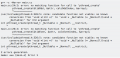# How to fix no matching function for call to 'pthread_create' in multithreading?

#### asilvester635

Joined Jan 26, 2017
73
Basically, I create an array with 10 elements inside main and create two threads. The first thread, calcOddIndex, will calculate the product of all elements with odd index inside the array. The second thread, calcEvenIndex, will calculate the product of all elements with even index inside the array. Main will finally output the results after two child threads finish.

It gives me the error below. I think I am not passing the array correctly to the pthread_create. How should I fix this?Here is my code:
Code:
#include <pthread.h>
#include <stdio.h>
#include <stdlib.h>

/* first thread will calculate the product of all elements with odd index inside the array */
void *calcOddIndex(int arr);
int oddIndexProduct = 1;
/* the second thread will calculate the product of all elements with even index inside the array */
void *calcEvenIndex(int arr);
int evenIndexProduct = 1;

int main() {

/* create an array with at least 10 elements */
int array[] = { 1, 2, 3, 4, 5, 6, 7, 8, 9, 10 };

/* get the default attributes */

/* create thread for calcOddIndex */
/* create thread for calcEvenIndex */

/* wait until both threads is done with their work */

// print the product of all elements with odd index
printf("product of odd index inside the array = %d\n", oddIndexProduct);
// print the product of all elements with even index
printf("product of even index inside the array = %d\n", evenIndexProduct);

} // end of main

/*
calculate the product of all elements with odd index inside the array
*/
void *calcOddIndex(int arr) {
for (int i=1; i<10; i=i+2) {
oddIndexProduct *= arr[i];
}
} // end of calcOddIndex

/*
calculate the product of all elements with even index inside the array
*/
void *calcEvenIndex(int arr) {
for (int i=0; i<10; i=i+2) {
evenIndexProduct *= arr[i];
}
} // end of calcEvenIndex

#### xox

Joined Sep 8, 2017
598
The callback function you're passing to pthread_create has the wrong prototype ("signature"). It has to be declared like this:

Code:
void* calcOddIndex(void* data);
Inside the function you cast the void* parameter "data" back to the correct type. In other words:

Code:
void* calcOddIndex(void* data){
int* arr = (int*)data;
for (int i=1; i<10; i=i+2) {
oddIndexProduct *= arr[i];
}
} // end of calcOddIndex